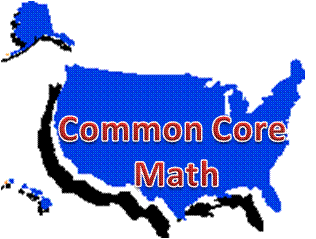# Common Core High School Geometry Teaching and Learning Resources

## Domain: MG: Modeling with Geometry• MG-A: Apply geometric concepts in modeling situations### MG-A: Apply geometric concepts in modeling situations

Standards:

• MG-A.1. Use geometric shapes, their measures, and their properties to describe objects (e.g., modeling a tree trunk or a human torso as a cylinder).
• MG-A.2. Apply concepts of density based on area and volume in modeling situations (e.g., persons per square mile, BTUs per cubic foot).
• MG-A.3. Apply geometric methods to solve design problems (e.g., designing an object or structure to satisfy physical constraints or minimize cost; working with typographic grid systems based on ratios).

Technology-enhanced investigations:

MIT BLOSSOMS: Video lessons with additional teacher and learner resources.  Descriptions are from the video summaries.

• Building Cryptosystems: "This video module presents an introduction to cryptography – the method of sending messages in such a way that only the intended recipients can understand them. In this very interactive lesson, students will build three different devices for cryptography and will learn how to encrypt and decrytp messages."  Aligns with MG-A.3.
• Engineering Innovation and Entrepreneurship: "This video lesson introduces students to the worlds of engineering innovation and entrepreneurship. ... The video will familiarize students with all the steps in the innovation process: from conception to launch. By the end of this lesson, students will be prepared for an optional long-term innovation project."  Aligns with MG-A.3.
• Using Geometry to Design Simple Machines: "This video is meant to be a fun, hands-on session that gets students to think hard about how machines work. It teaches them the connection between the geometry that they study and the kinematics that engineers use -- explaining that kinematics is simply geometry in motion. In this lesson, geometry will be used in a way that students are not used to."  Aligns with MG-A.3.

National Math and Science Initiative: Lesson: Using Linear Equations to Define Geometric Solids: Use this lesson for geometry within a unit on volume applications.  Students write the equations of lines and graph linear equations that define bounded regions, calculate the areas and perimeters of the regions, revolve the planar regions about horizontal and vertical lines to create solids, and calculate the volumes of the resulting solids; they use technology (virtual manipulatives) to create and revolve planar figures about horizontal and vertical lines and model the conceptual understanding using a real world situation.  Targeted standards include GMD-A.3, GMD-B.4, GPE-B.7 and MG-A.1.  Common Core Math Practice standards 1-7 are also addressed.

NCTM Illuminations: Geometric Solids: Use this virtual manipulative to "learn about various geometric solids and their properties. You can manipulate and color each shape to explore the number of faces, edges, and vertices."  View the solid or its net. You can also use this tool to help determine the relationship between the number faces, vertices, and edges.

Wolfram Demonstrations Project: Download the free Wolfram CDF player to interact with the following manipulatives:

Multiple Choice:

Khan Academy: Volume and Surface Area unit. Practice questions with videos:

Constructed-response:

Illustrative Mathematics: Geometry:

Mathematics Assessment Project: High School: Geometry:

Mathematics Vision Project, Secondary 3 Student Edition:

• Module 5: Modeling with Geometry: This module contains 8 classroom tasks.  Multiple standards are addressed. Standards MG-A.1 is found in task 3; MG-A.1, MG-A.2, MG-A.3 are found in task 4.